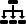Neural Networks with Python on the Web

 Filter by NN Type Feedforward NN Reccurent NN Deep Belief Network Convolutional Neural Network Recurrent neural network toolbox for Python and Matlab LSTM Recurrent Neural Network Convolutional Neural Network and RNN MxNET ADAPTIVE LINEAR NEURON (Adaline) neural network library for python Generative Adversarial Networks (GAN) Spiking Neural Netorks (SNN) Self-Organising Maps (SOM) TensorFlow Neural Network Multi-Layer Perceptron Echo Recurrent Neural Network CNN, RNN RCNN TensorFlow encoder/decoder FFNN and CNN Softmax Classifier ShallowLearn Bi-directional LSTM Skip-Gram Model auto-encoder Sequential Bayesian Neural Networks FCNNML Sandbox - Online Machine Learning Algorithms